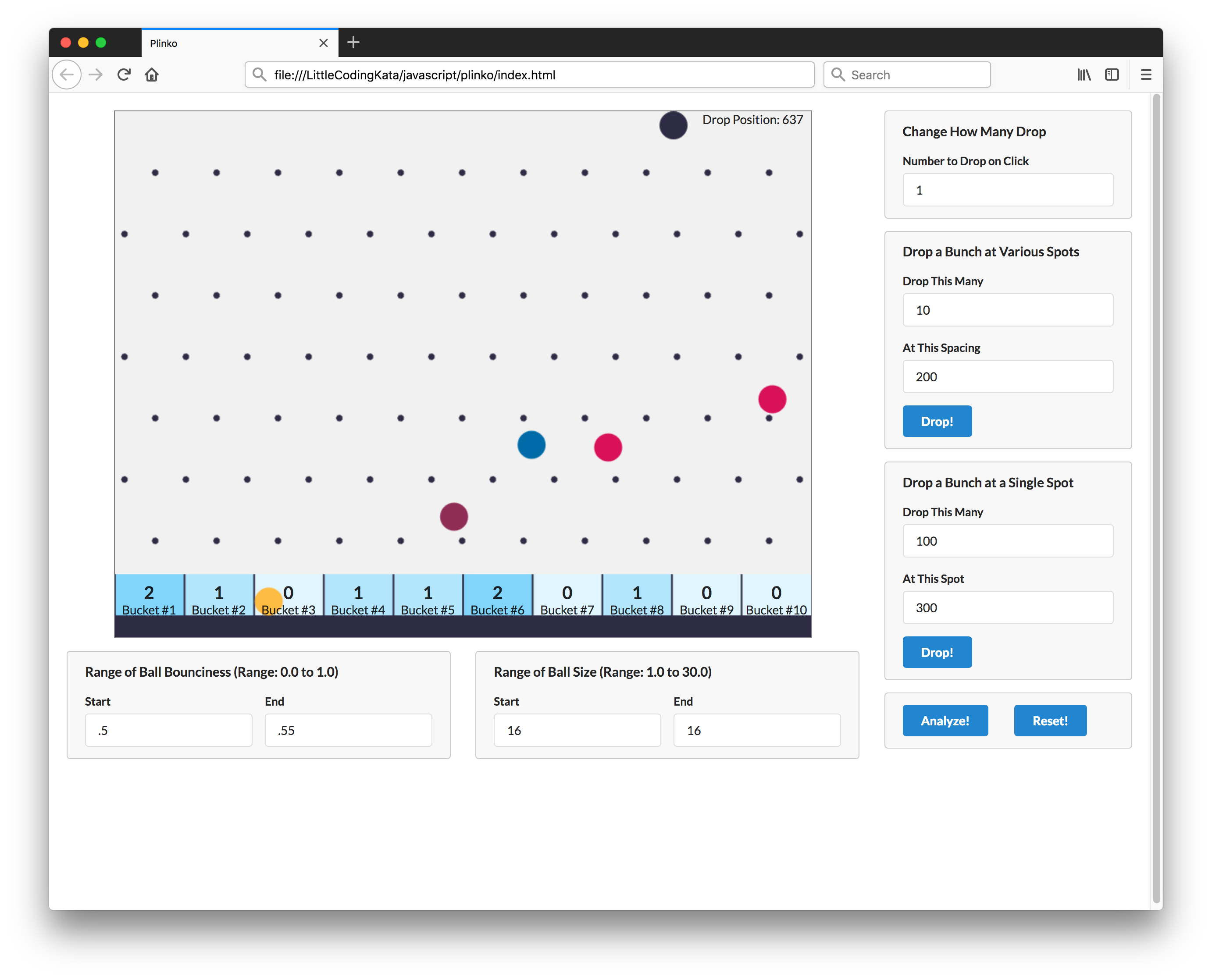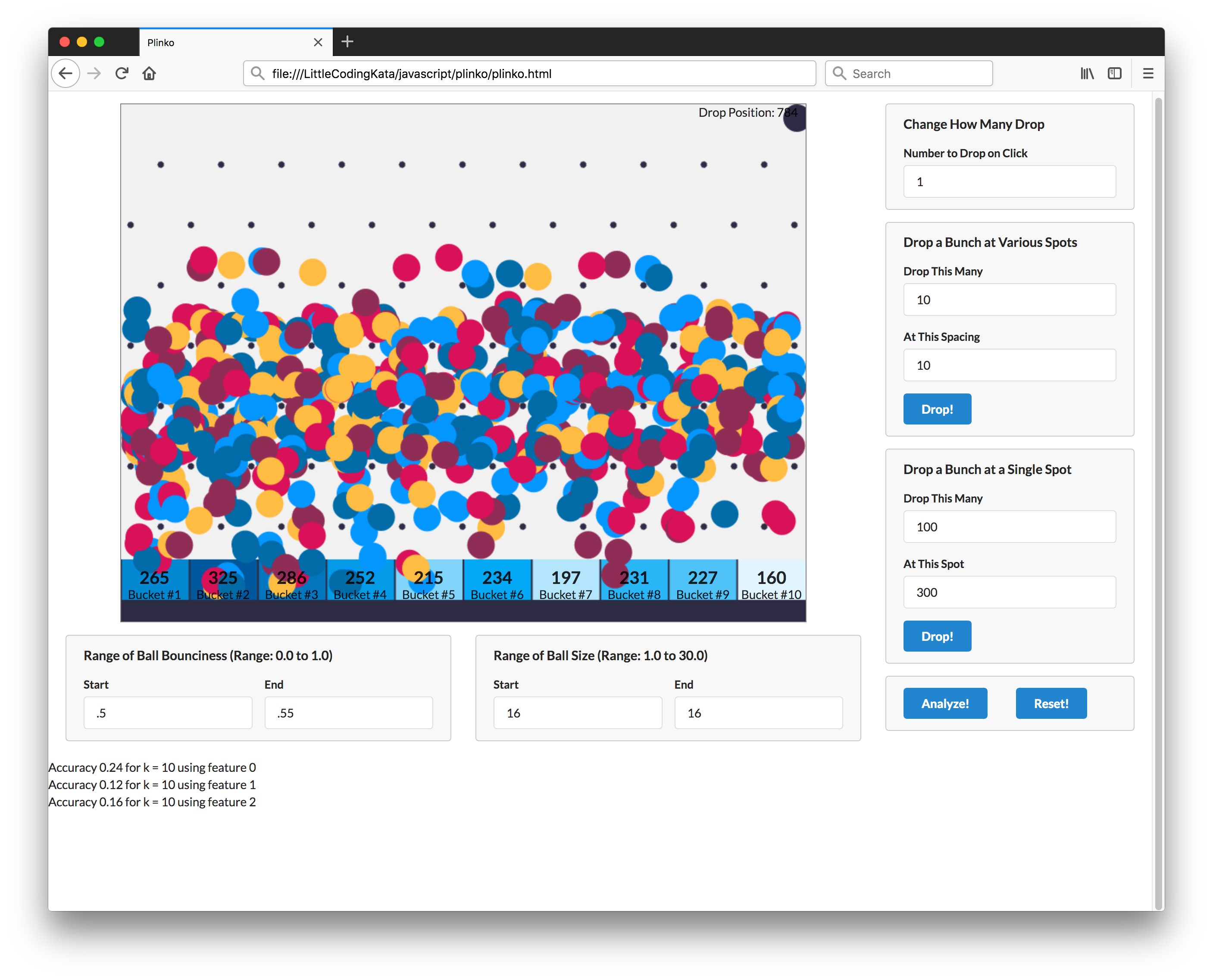### Project Notes

A demonstration of the k-nearest neighbors algorithm implemented in javascript in a browser.

This is my working of an example from the Machine Learning with Javascript course.

Plinko is a demonstration of the k-nearest neighbors algorithm implemented in javascript in a browser.

## Problem

Plinko is a simple game (like a pachinko machine). The problem to be solved: Given where ball is dropped from, can we predict which bucket it will land in?

The dependent variable (label) is the bucket. This is a classification problem as label values belong to discrete set.

The independent variables (features) that may be relevant include:

• drop position
• ball bounciness
• ball size

## How K-Nearest Neighbor Works

• given a prediction point (set of features to predict label for)
• collect sample data
• for each observation, calculate the feature distance from prediction point
• sort and take top ‘k’ records
• select the most common label
• that is the prediction

## Multi-Dimensional KNN

When modeling with multiple features, consider:

• normalize or standardise the features

### Feature Normalization

• normalize - 0..1
• standardize - normal standard deviation around 0

Normalising with MinMax

``````normalized = (value - min) / (max - min)
``````

## Feature Selection

• change in drop position - has predictable impact on bucket
• change in bounciness - has impact on bucket, but not predictably

## Running the Demonstration

See plinko.html or locally `open plinko.html`

Drop enough balls to make a sample set

• optionally set bounciness and size rangeClick the analyze button. Results inserted at the bottom of the page: